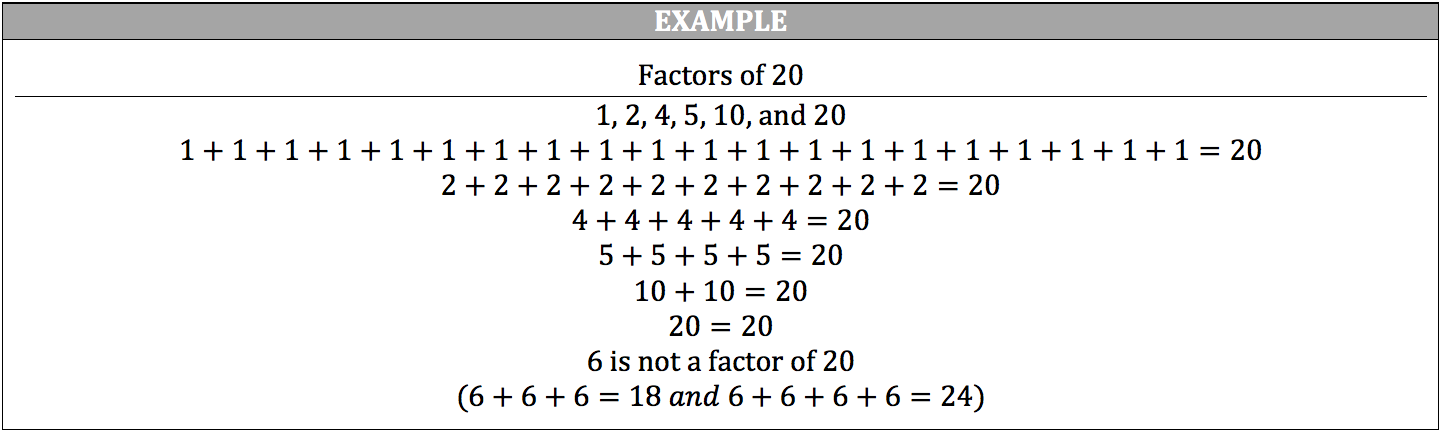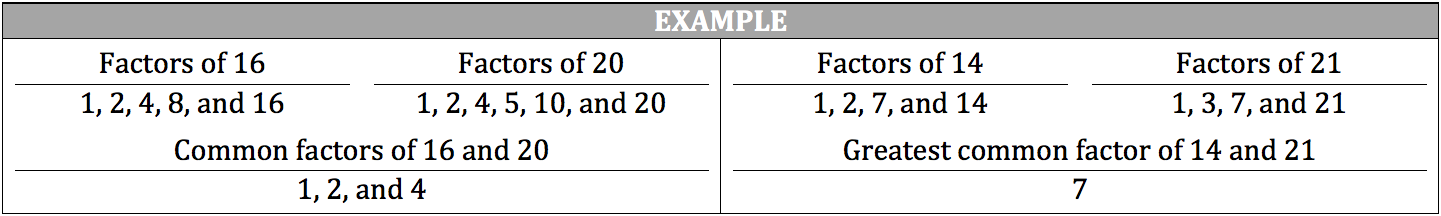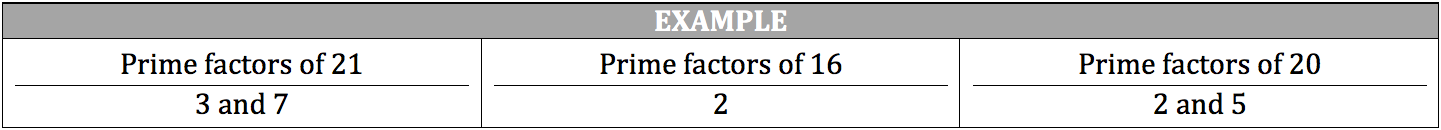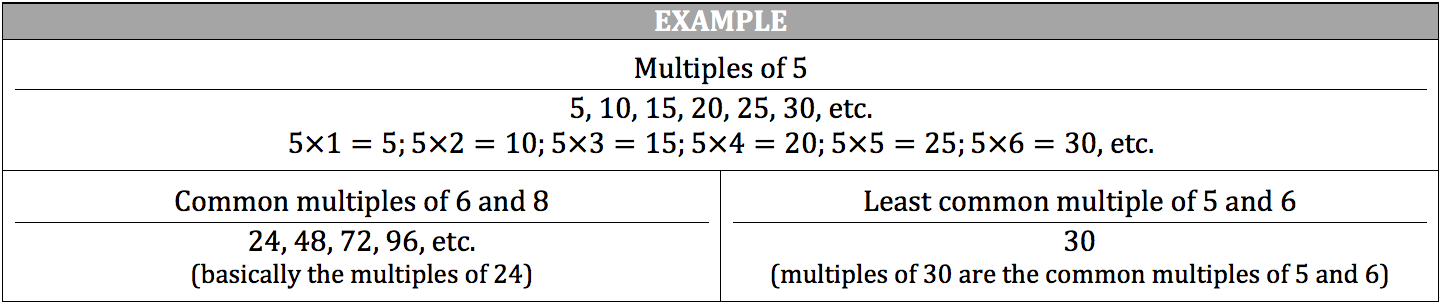The factors of a number are integers that can be divided evenly (without a remainder) into the number. For example, the number 20 is divisible by 5 in that 5 can go into 20 four times without going over or under the number 20. However, the number 20 is not divisible by 6, as 6 can only go into the number 20 three times before going over.Common factors are factors that two or more numbers have in common, such as 4 is a common factor of 16 and 20. The greatest common factor of two or more numbers is the largest common factor shared between numbers, such as 7 is the greatest common factor of 14 and 21.Prime factors are the factors of a number that are prime numbers. 1 is NOT a prime factor.The multiples of a given number are those numbers that can be divided by that given number without a remainder. You can find the multiples of a number by multiplying it by another number, such as 2 1 2 and 2 2 4. Thus, 2 and 4 are multiples of 2. Incidentally, if the sum of the digits of a number is divisible by 3, then the number is a multiple of 3 (ex. 561→5 6 1 12). Any number that is a multiple of all the given numbers is called a common multiple. The least common multiple is the smallest multiple of two or more numbers, such as 24 is the least common multiple of 8 and 12.